# 3. [-12 Points] DETAILS ZILLDIFFEQMODAP115.2.011. Find the eigenvalues in and eigenfunctions yn(x) for the given boundary-value...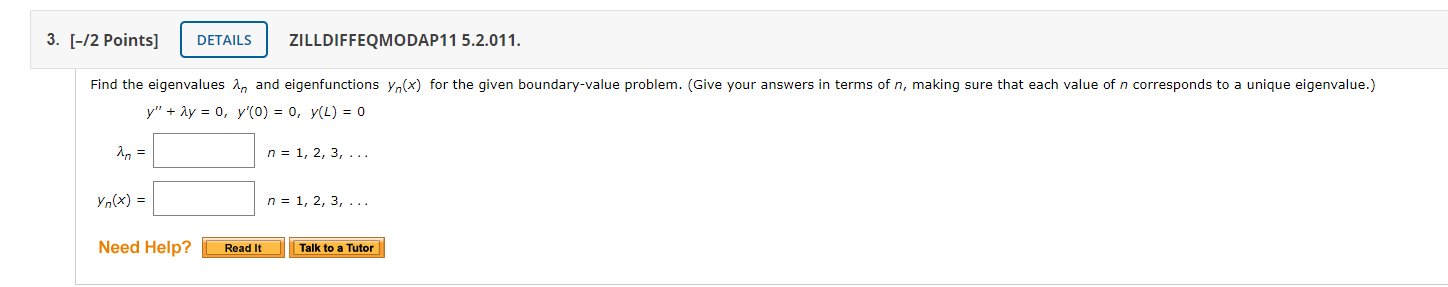3. [-12 Points] DETAILS ZILLDIFFEQMODAP115.2.011. Find the eigenvalues in and eigenfunctions yn(x) for the given boundary-value problem. (Give your answers in terms of n, making sure that each value of n corresponds to a unique eigenvalue.) y" + 2y = 0, y'O) = 0, y(L) = 0 in = n = 1, 2, 3, ... yn(x) = n = 1, 2, 3, ... Need Help? Read It Talk to a Tutor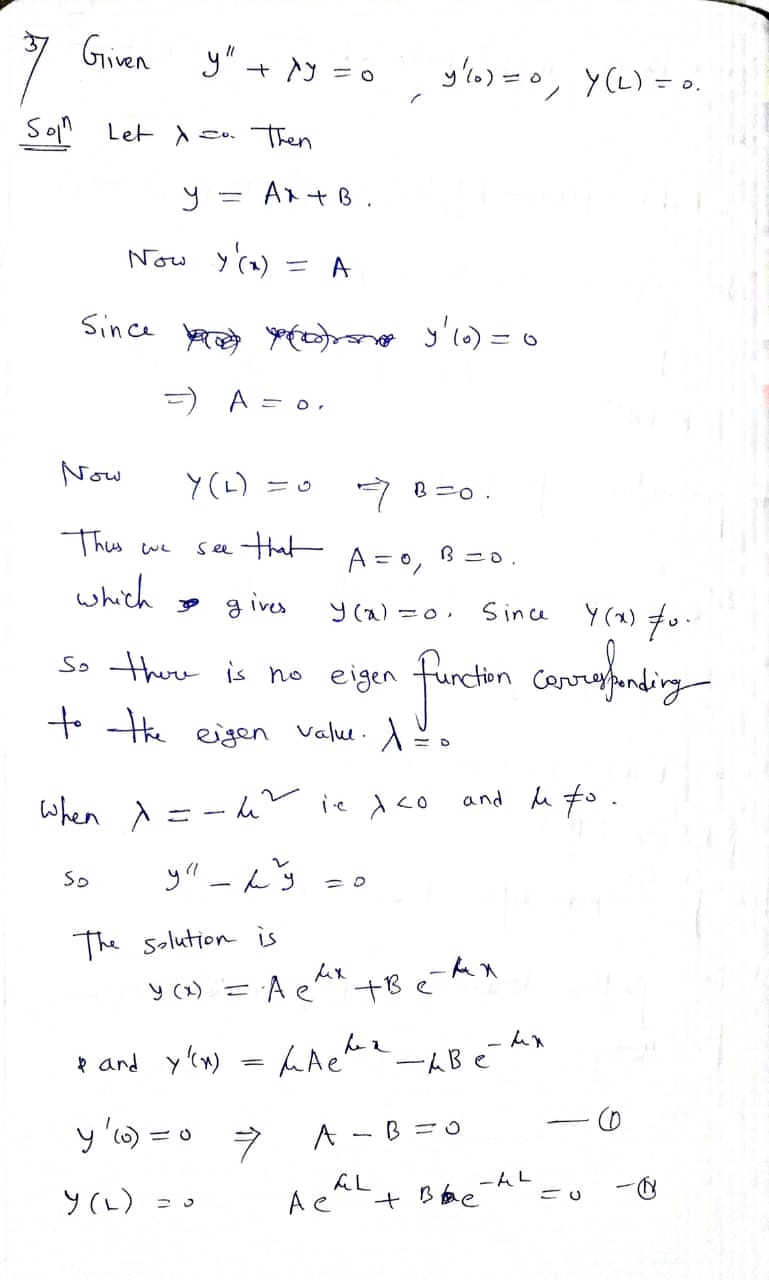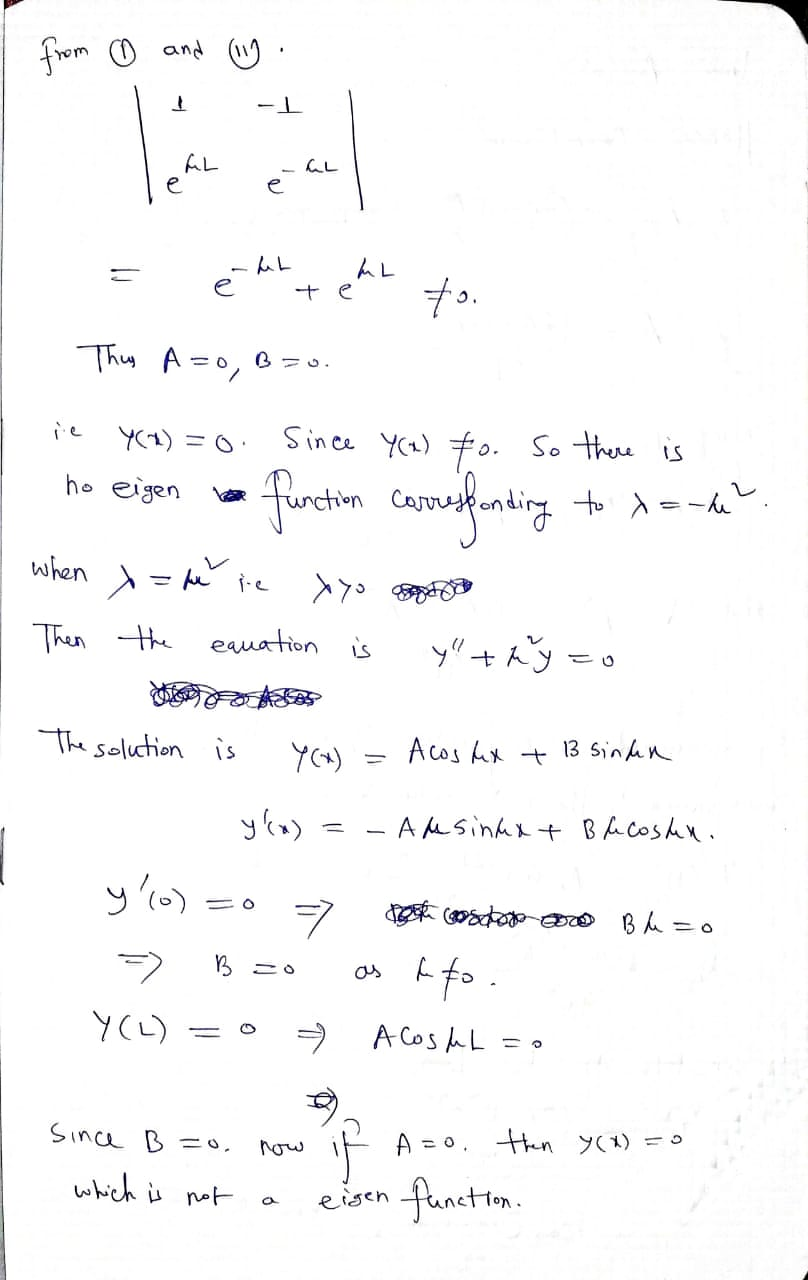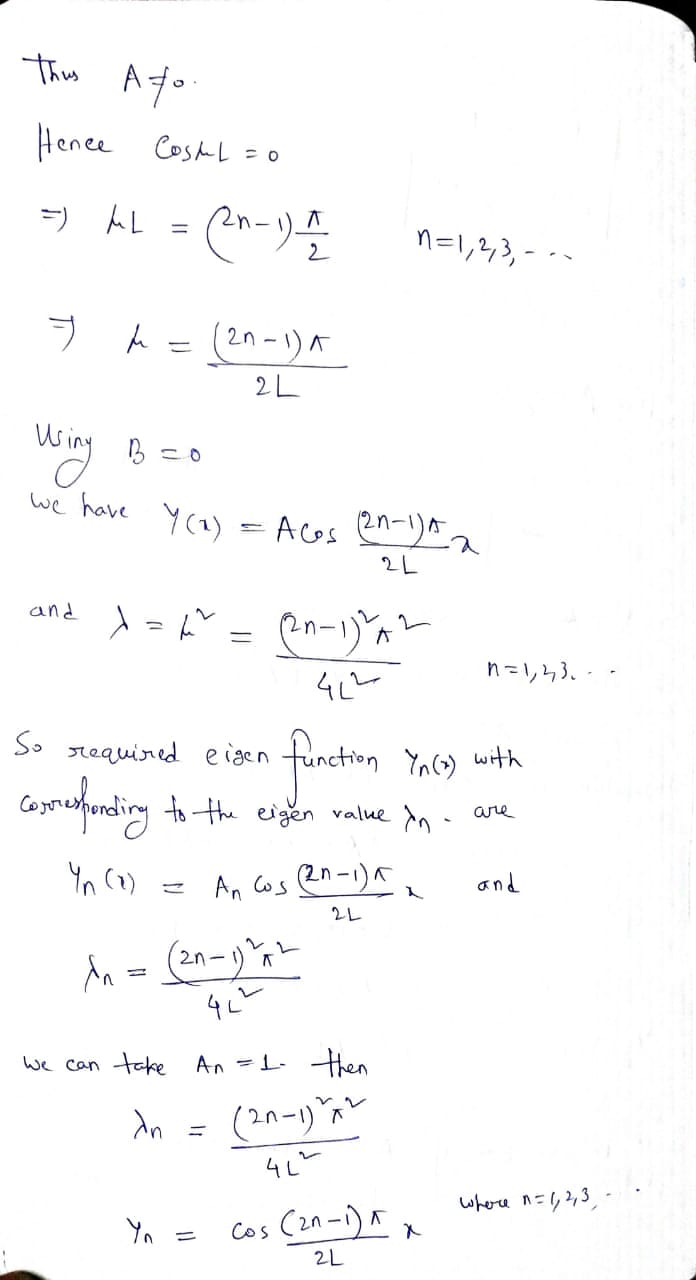##### Add Answer of: 3. [-12 Points] DETAILS ZILLDIFFEQMODAP115.2.011. Find the eigenvalues in and eigenfunctions yn(x) for the given boundary-value...
Similar Homework Help Questions
• ### 2. [-12 Points) DETAILS ZILLDIFFEQMODAP11 5.2.009. Find the eigenvalues in and eigenfunctions yn(x) for the given...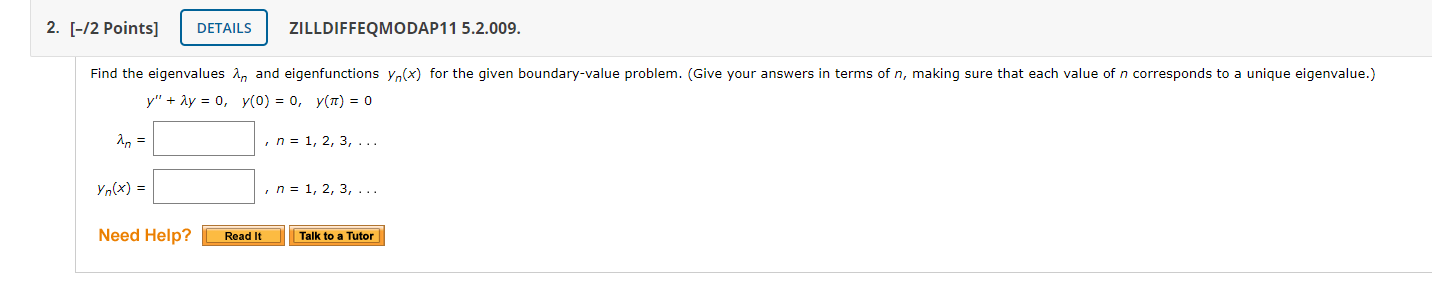2. [-12 Points) DETAILS ZILLDIFFEQMODAP11 5.2.009. Find the eigenvalues in and eigenfunctions yn(x) for the given boundary-value problem. (Give your answers in terms of n, making sure that each value of n corresponds to a unique eigenvalue.) y" + 2y = 0, y(0) = 0, y() = 0 , n = 1, 2, 3, ... yn(x) = in = 1, 2, 3, ... Need Help? Read It Talk to a Tutor

• ### 4. [-/2 Points] DETAILS ZILLDIFFEQMODAP11 5.2.013. Find the eigenvalues in and eigenfunctions yn(x) for the given...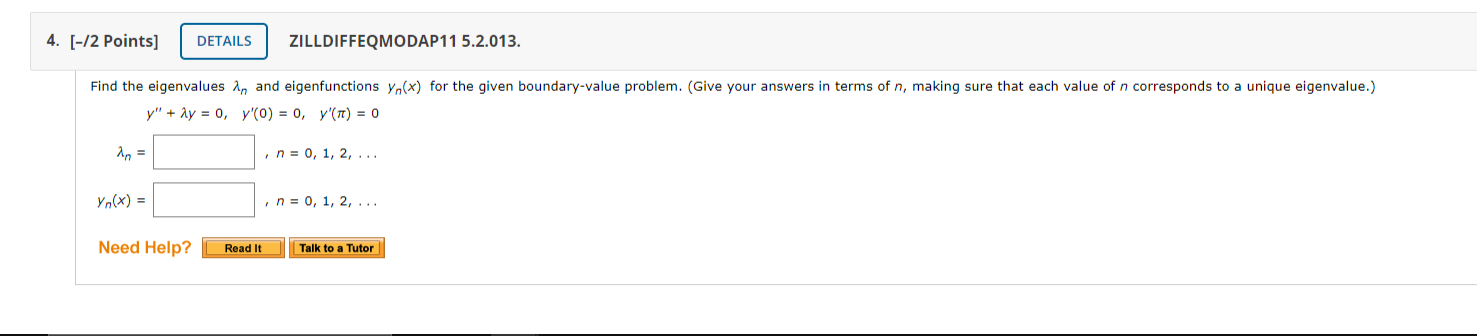4. [-/2 Points] DETAILS ZILLDIFFEQMODAP11 5.2.013. Find the eigenvalues in and eigenfunctions yn(x) for the given boundary-value problem. (Give your answers in terms of n, making sure that each value of n corresponds to a unique eigenvalue.) y" + 2y = 0, y (O) = 0, y'(TT) = 0 in = , n = 0, 1, 2, ... Yn(x) = , n = 0, 1, 2, ... Need Help? Read It Talk to a Tutor

• ### 5. [-12 Points) DETAILS ZILLDIFFEQMODAP11 5.2.015. Find the eigenvalues in and eigenfunctions yn(x) for the given...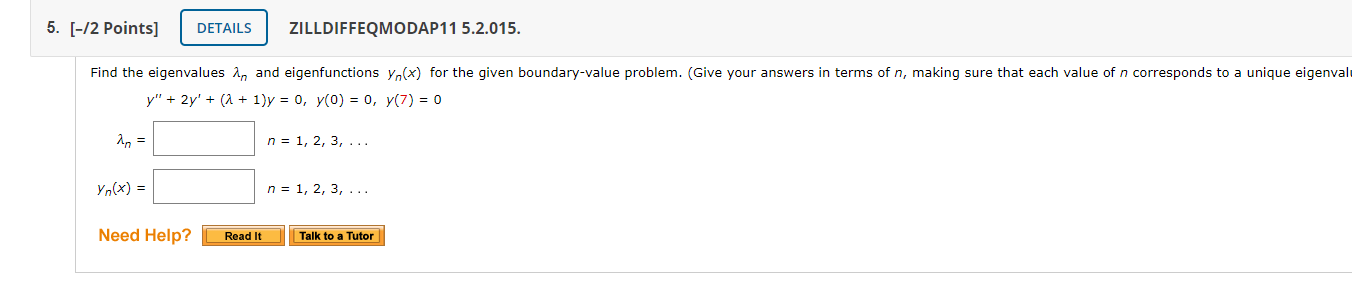5. [-12 Points) DETAILS ZILLDIFFEQMODAP11 5.2.015. Find the eigenvalues in and eigenfunctions yn(x) for the given boundary-value problem. (Give your answers in terms of n, making sure that each value of n corresponds to a unique eigenval y" + 2y' + (1 + 1)y = 0, y(0) = 0, y(7) = 0 n = 1, 2, 3, ... yn(x) = n = 1, 2, 3, ... Need Help? Read It Talk to a Tutor

• ### 6. (-/2 points) DETAILS ZILLDIFFEQMODAP11 5.2.017. Find the eigenvalues in and eigenfunctions yn(x) for the given...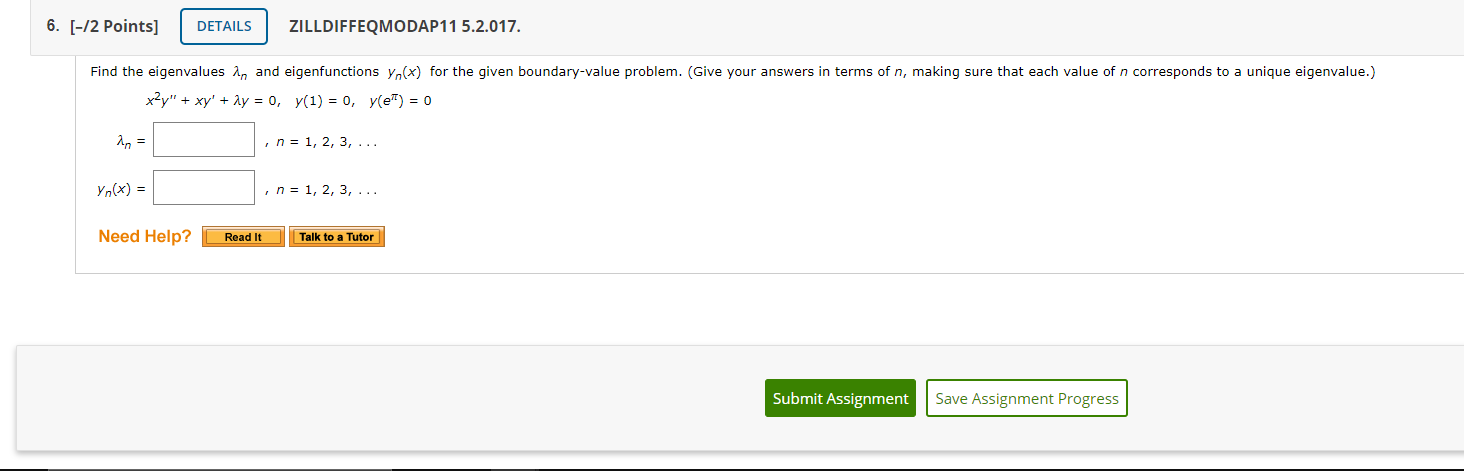6. (-/2 points) DETAILS ZILLDIFFEQMODAP11 5.2.017. Find the eigenvalues in and eigenfunctions yn(x) for the given boundary-value problem. (Give your answers in terms of n, making sure that each value of n corresponds to a unique eigenvalue.) x2y" + xy' + 2y = 0, y(1) = 0, y(e) = 0 in = , n = 1, 2, 3, ... yo(x) = , n = 1, 2, 3, ... Need Help? Read It Talk to a Tutor Submit Assignment Save Assignment...

• ### Find the eigenvalues in and eigenfunctions yn(x) for the given boundary-value problem. (Give your answers in...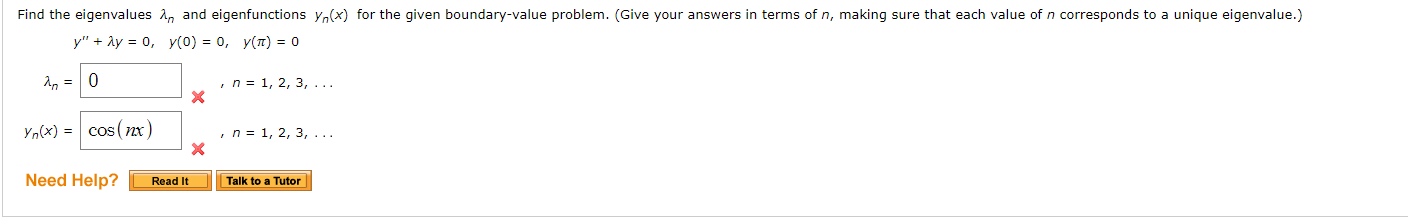Find the eigenvalues in and eigenfunctions yn(x) for the given boundary-value problem. (Give your answers in terms of n, making sure that each value of n corresponds to a unique eigenvalue.) y" + y = 0, y(0) = 0, y(t) = 0 in = 1, 2, 3, ... în=0 Yn(x) = cos(nx) , n = 1, 2, 3, ... Need Help? Read It Talk to a Tutor

• ### and 3. Find the eigenvalues and eigenfunctions for the given boundary-value problem. There are 3 cases...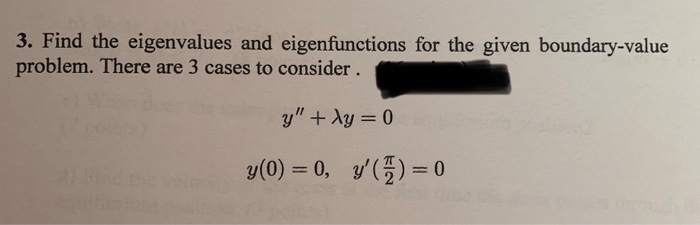and 3. Find the eigenvalues and eigenfunctions for the given boundary-value problem. There are 3 cases to consider. g" + Ag = 0 y(0) = 0, y'(%) = 0 8. Given the initial value problem (3 – 4 g" + 2z +174 = In , g(3) = 1, y'(3) = 0, use the Existence and Uniqueness Theorem to find the LARGEST interval for which the problem would have a unique solution. Show work.

• ### (1 point) Determine the values of (eigenvalues) for which the boundary-value problem g” + y =...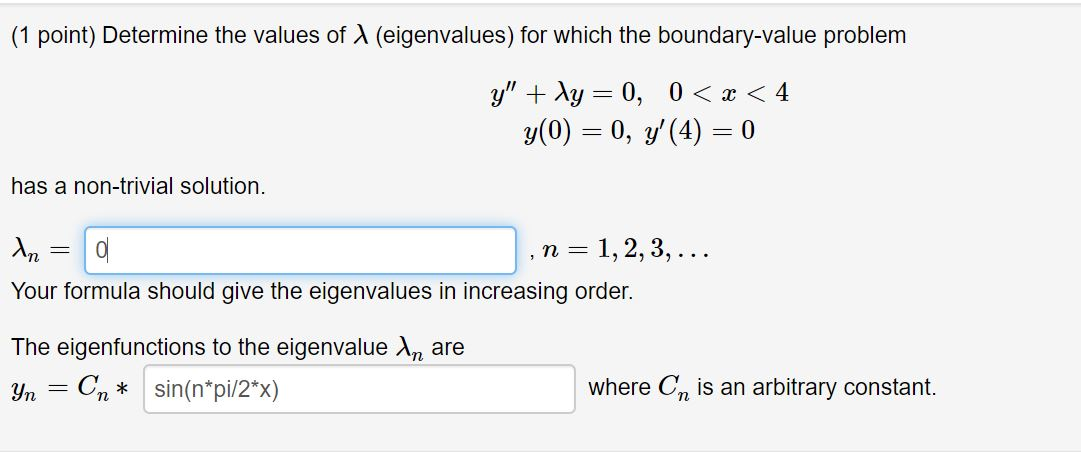(1 point) Determine the values of (eigenvalues) for which the boundary-value problem g” + y = 0, 0 < x < 4 y(0) = 0, y' (4) = 0 has a non-trivial solution. An = a , n=1,2,3,... Your formula should give the eigenvalues in increasing order. The eigenfunctions to the eigenvalue in are Yn = Cn* sin(n*pi/2*x) where On is an arbitrary constant.

• ### Boundary Value Problem with Eigenvalues

Find the eigenvalues (λn)^2 and eigenfunctions yn(x) of the following boundary value problem.yʺ+λ2y=0yʹ(0)=0y(6π)=0λn2=yn(x)=

• ### Find the eigenvalues and eigenfunctions for the differential operator L(y)=−y″L(y)=−y″ with bound...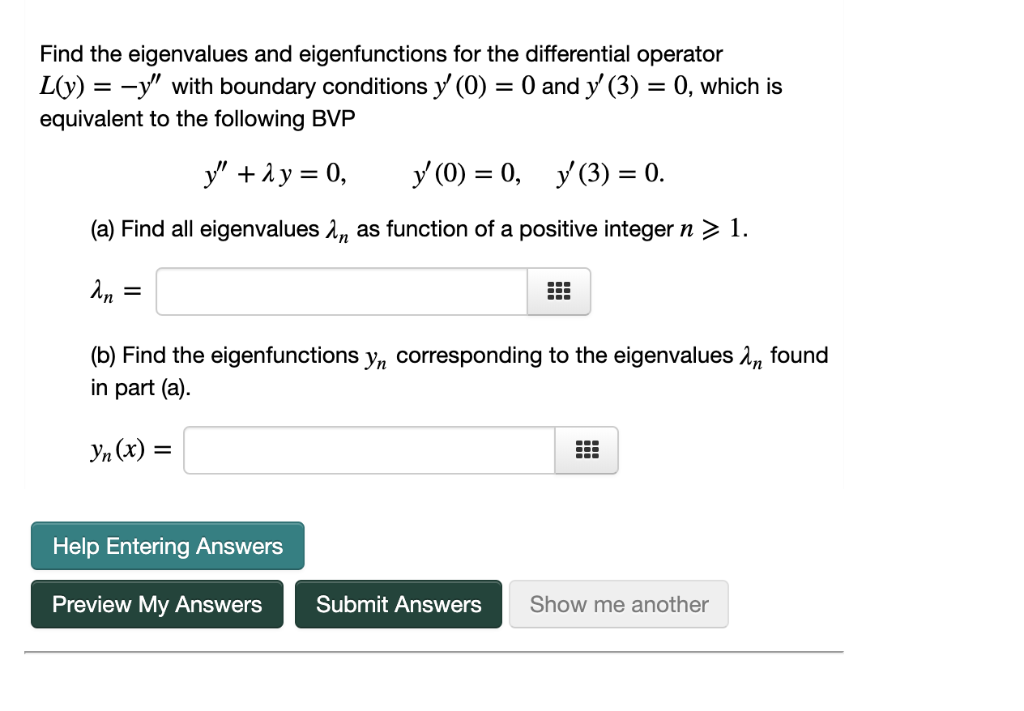Find the eigenvalues and eigenfunctions for the differential operator L(y)=−y″L(y)=−y″ with boundary conditions y′(0)=0y′(0)=0 and y′(3)=0y′(3)=0, which is equivalent to the following BVP y″+λy=0,y′(0)=0,y′(3)=0.y″+λy=0,y′(0)=0,y′(3)=0. Find the eigenvalues and eigenfunctions for the differential operator L(y)--y" with boundary conditions y (0)0 and y' (3)-0, which is equivalent to the following BVP (a) Find all eigenvalues 2n as function of a positive integer n > 1. (b) Find the eigenfunctions yn corresponding to the eigenvaluesn found in part (a). Help Entering Answers ew...

• ### Find the eigenvalues and eigenfunctions for the boundary value problem, 2x 2 y 00 + 2xy 0 + λy = ...

Find the eigenvalues and eigenfunctions for the boundary value problem, 2x 2 y 00 + 2xy 0 + λy = 0, y(1) = 0, y 0 (2) = 0.

Free Homework App